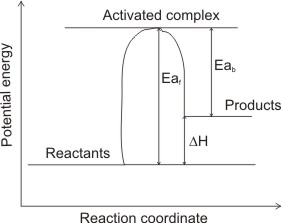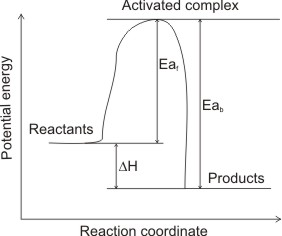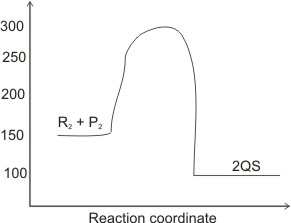## Use of Energy Level Diagrams

During chemical reactions, reactant molecules do not form products directly, but absorb energy and collide with one another to form temporarily complex, high energy molecules.

These molecules, also called activated complex are less stable than either the reactants or the products and can therefore either break into products (forward path) or back into the reactants (backward path). They place an energy barrier (called activation energy) between reactants and products.

What is Activation Energy?

Activation energy is the minimum energy necessary for reactions to occur. For reactions to take place therefore, the colliding molecules must absorb enough energy to overcome the energy barrier (i.e. activation energy).

Notice that it isn’t all collisions that lead to reactions, it is only those which have the same or higher energies than the activation energy that will result in reactions. Therefore, only a small fraction of the colliding molecules will react.

Energy level diagrams illustrate the above discuss.(1) Endothermic(2) Exothermic

From the diagrams above, the heat of reactions ∆H can be deduced. Eaf represents the activation energy of the forward reaction; Eab represents the activation energy of the backward reaction (in which the products of the forward reaction become the reactants.

Enthalpy change is the difference between Eaf and Eab.

I.e., ∆H = Eaf - Eab.

In figure (1), the reactants are at lower total energy than the products (Eaf > Eab) hence, to balance the energy, heat energy is absorbed by the system (to be able to reach the energy level of the products) – the reaction is endothermic (∆H = +ve).

In fig (2), the reactants are at higher total energy than the products (Eaf < Eab). This means that, to balance the energy, excess of energy has to be released as heat to the surroundings - the reaction is exothermic (∆H = - ve).

From the energy level (or energy profile) diagrams above: Note:

Activation energy, Ea is the energy difference between the activated complex and energy of reactants. It is the energy required by reactant molecules to become activated complex, hence it is always endothermic.

The heat of reaction (or enthalpy change, ∆H) is the difference between the energy levels of products and reactants.

I.e., Energy of products - Energy of reactants. Or Eaf - Eab. If energy of products and Eaf are greater than energy of reactants and Eab, ∆H is positive, i.e., the reaction is endothermic.

But if energy of products and Eaf are less than energy of reactants and Eab, then ∆H is negative, i.e., the reaction is exothermic.

CalculationsIn the diagram above, determine the (i). activation energy (ii). activation energy for the backward reaction (iii). enthalpy of the reaction.

Solution

Notice that when a question states activation energy, without indicating whether it is for the forward or backward reaction, it should be taken for the forward reaction.

(i). The activation energy is for the forward reaction. This is the energy difference between the activated complex and the reactants. I.e., 300 - 150 = 150 kJ/mol

(ii). Here, the reactants are QS I.e., 300 - 100 = 200 kJ/mol

(iii). The enthalpy of the reaction is the energy difference between the products and the reactants.

I.e., 100-150 = - 50 kJ/mol.

Notice that the enthalpy is a -ve value, meaning that the reaction is exothermic.

 Like This Post? Please Share!!!!

Copyright , All Rights Reserved Free Chemistry Online | About Us | Usage of Content | Total Disclosures | Privacy Policy